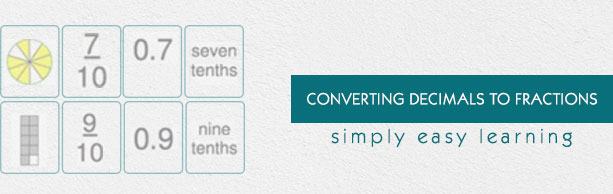# Converting Decimals to Fractions

This tutorial provides comprehensive coverage of converting decimals to fractions based on Common Core (CCSS) and State Standards and its prerequisites. Students can navigate learning paths based on their level of readiness. Institutional users may customize the scope and sequence to meet curricular needs. This simple tutorial uses appropriate examples to help you understand converting decimals to fractions in a general and quick way.

# Audience

This tutorial has been prepared for beginners to help them understand the basics of converting decimals to fractions. After completing this tutorial, you will find yourself at a moderate level of expertise in converting decimals to fractions, from where you can advance further.

# Prerequisites

Before proceeding with this tutorial, you need a basic knowledge of elementary math concepts such as number sense, addition, subtraction, multiplication, division, whole numbers, fractions, types of fractions, decimals, comparing and ordering whole numbers and so on.